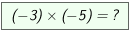Integers ... multiply -3- Two negative numbers are given. With the sliders factor1 and factor2 you can change the factors. You can let the program show you step by step how to multiply two negative numbers. Click several times on  ? »  If you have understood this, you can solve some problems.